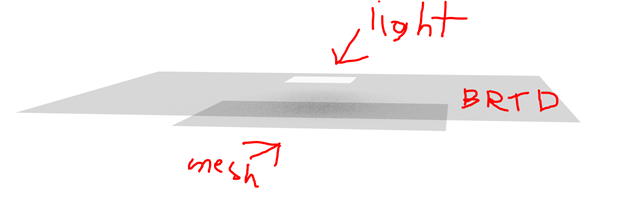# Unexpected Asymmetric BRTD Behavior (Post Has Pictures)

Hello all,

Question: Why does my object interact with the simulated rays at all?

Background: I want to create a material that allows perfect specular transmission in one direction and no transmission or reflection in the other direction. I built the following BRTD function and .cal file for this purpose:

BRTDfunc
void BRTDfunc testing
10 refl_red refl_grn refl_blu trans_red trans_grn trans_blu 0 0 0 testingBRTDf.cal
0
9 0 0 0 0 0 0 0 0 0

testingBRTDf.cal
(Note in file “(x)” is just one of these symbols >>> *)
refl_red = if(Nz, 0 (x) RdotP, 0 (x) RdotP);
refl_grn = if(Nz, 0 (x) RdotP, 0 (x) RdotP);
refl_blu = if(Nz, 0 (x) RdotP, 0 (x) RdotP);
trans_red = if(Nz, 1 (x) RdotP, 0 (x) RdotP);
trans_grn = if(Nz, 1 (x) RdotP, 0 (x) RdotP);
trans_blu = if(Nz, 1 (x) RdotP, 0 (x) RdotP);

To validate this material I rendered the following:And this resulted in the following test mesh results:

However, when I ran the simulation without the material present I got results roughly 15% higher:

Since any rays that pass through the material should only receive a factor of 1, why does the presence of the material reduce the energy on the test mesh by 15% and how can I remove this behavior?

Prior to posting my original post, I tried to solve this problem by repeatedly rerunning the model to empirically find a factor that would compensate for this roughly 15% energy loss. Using the test mesh maximum as the metric to match, this rerun method gave me a value of 1.1722, which I then implemented into testingBRTDf.cal as follows:

refl_red = if(Nz, 0xRdotP, 0xRdotP);
refl_grn = if(Nz, 0xRdotP, 0xRdotP);
refl_blu = if(Nz, 0xRdotP, 0xRdotP);
trans_red = if(Nz, 1.1722xRdotP, 0xRdotP);
trans_grn = if(Nz, 1.1722xRdotP, 0xRdotP);
trans_blu = if(Nz, 1.1722xRdotP, 0xRdotP);

However, after re-running the simulation with the testingBRTDf.cal delevoped through model reruns, I compared its test mesh results to that of the model without the object. The results of the two test meshes did not match, and I suspect that the root cause of both this solution and the problem statement of my original post stem from a similar underlying issue.

I think the problem is that you should not be using the RdotP factor. Specular transmission and reflection are specified as a fraction from 0-1, and should not include a cosine factor.

Ah I see, much thanks! My error was that I interpreted RdotP as the variable that contained the scalar value of the intensity of a ray. Stated another way, I had thought I was writing some form of the following:

int RdotP;
if(Nz >= 0)
{
RdotP = RdotP*1;
}
else
{
RdotP = 0
}

Per my problem, the following solution works:

refl_red = if(Nz, 0, 0);
refl_grn = if(Nz, 0, 0);
refl_blu = if(Nz, 0, 0);
trans_red = if(Nz, 1, 0);
trans_grn = if(Nz, 1, 0);
trans_blu = if(Nz, 1, 0);